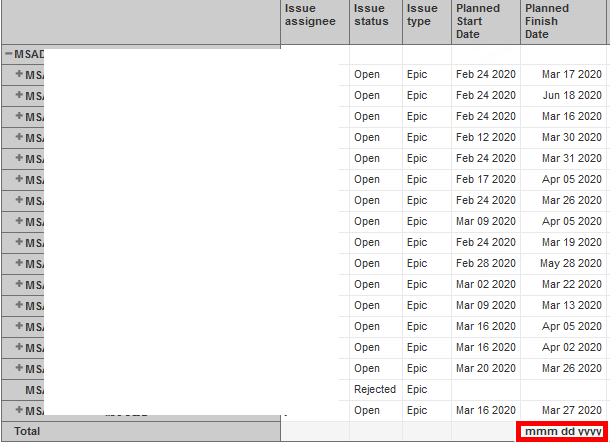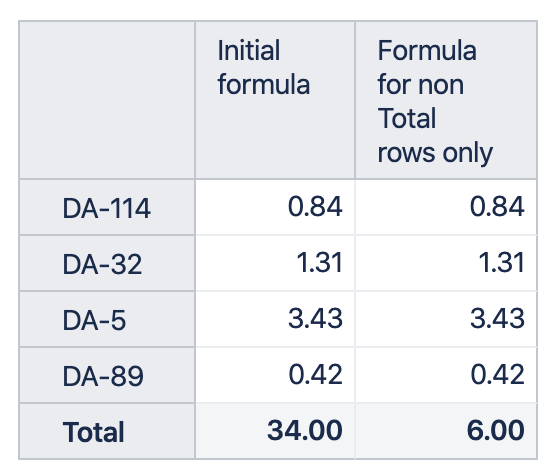# How to customize the “Total” option with my defined formulation

I add the “Total” option in my report, but it seems just add the number, without meaningful calculation. May I customize the “Total” option with my defined formulation.

eazyBI applies the same formula on Totaling row as on any other row. It seems it can’t apply the same formula on Totaling row and it gives empty results.

If a formula gets empty results on Total row, eazyBI forces to sum values of any row in this column. Current result is a sum of rows.

Please take into account, properties, for example, issue properties does not have any values on Total row. Total row can treat measures and formulas differently than regular rows.

You can access total row and apply a different formula there. You can address row member by name `\$total_aggregate`.

With the eazyBI version 5.0 or higher you can address the total row and any other rows with this formula:

``````CASE
WHEN CurrentTuple(VisibleRowsSet()).Item(0).Name = '\$total_aggregate'
THEN
-- total row calculation
ELSE
-- calculation on any other row
END
``````

Daina / support@eazybi.com

Thank you very much for your solution, it works for me, I could set some specific formula as I wish in total row now. However another issue occurred, I want to count the non-blank date number of [Planned Finish Date] column and set it into total row, but it seem the counted result can’t be set as numberic type. Do you have any solution about it. Thank you.It is great my suggestion to address the total line was helpful.

While there is an option to apply a different formula for total line, eazyBI does not have an option to set formatting for total row explicitly. Currently, we expect to have the same data type for any scenario within one measure and therefore there are no plans to change this behavior.

I would suggest adding a new measure for counting issues with those dates.

Daina / support@eazybi.com

Hi

Can you please elaborate further on how you access the “total row” formula.

I tried adding the formula to my existing calculated measure but I keep getting errors. How would i add it to this formula to ensure the total row only calculates the values displayed in the report:

Case when [Measures].[Duration of Task (Week)]>=1 then
([Measures].[Original estimated hours with target end]/
[Measures].[Original estimated hours with target end]
END

Duration of task (week) is a calculated formula based on the days between target start and target end

Duration of Task is based on Issue properties (target start and target end dates). Therefore, it has empty value for Total and the ELSE scenario ([Measures].[Original estimated hours with target end] for any issue) is applied to Total.

You can use the formula I shared in the first reply and apply the whole formula only for non total rows. This will make empty value for Total row and will force to SUM values on rows for Total row:

``````CASE
WHEN CurrentTuple(VisibleRowsSet()).Item(0).Name <> '\$total_aggregate'
THEN
Case when [Measures].[Duration of Task (Week)]>=1 then
([Measures].[Original estimated hours with target end]/